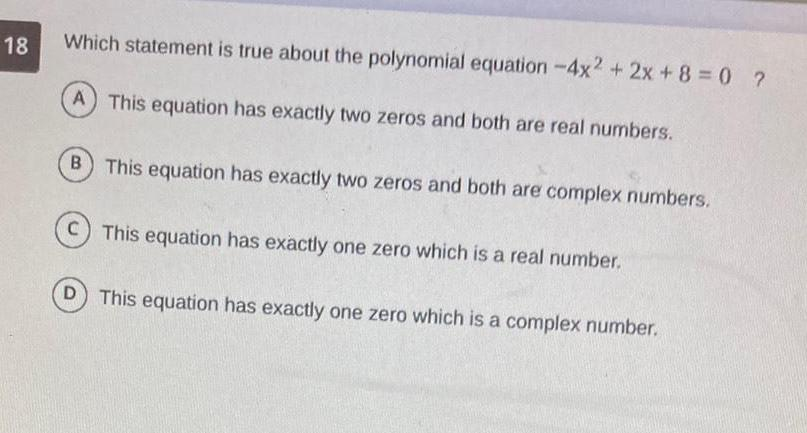Question:

# 18 Which statement is true about the polynomial equation 4x2

Last updated: 3/9/202318 Which statement is true about the polynomial equation 4x2 2x 8 0 A This equation has exactly two zeros and both are real numbers B This equation has exactly two zeros and both are complex numbers C This equation has exactly one zero which is a real number D This equation has exactly one zero which is a complex number